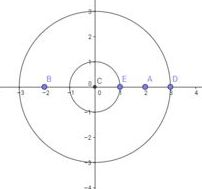# Just like Gianlino's Disc. Only 1000 times easier

Geometry Level 5Points $A$ and $B$ are placed at $(2,0)$ and $(-2,0)$ respectively. A circle of radius 3 is then drawn centered at the origin.

Next, a point $E$ is randomly placed on the circumference of circle C centered at the origin with radius 1.

If the probability that the circumcenter of $\triangle ABE$ lies within the circle of radius 3 can be represented by $\dfrac{m}{n}$ where $m,n$ are coprime positive integers, find m+n.

×